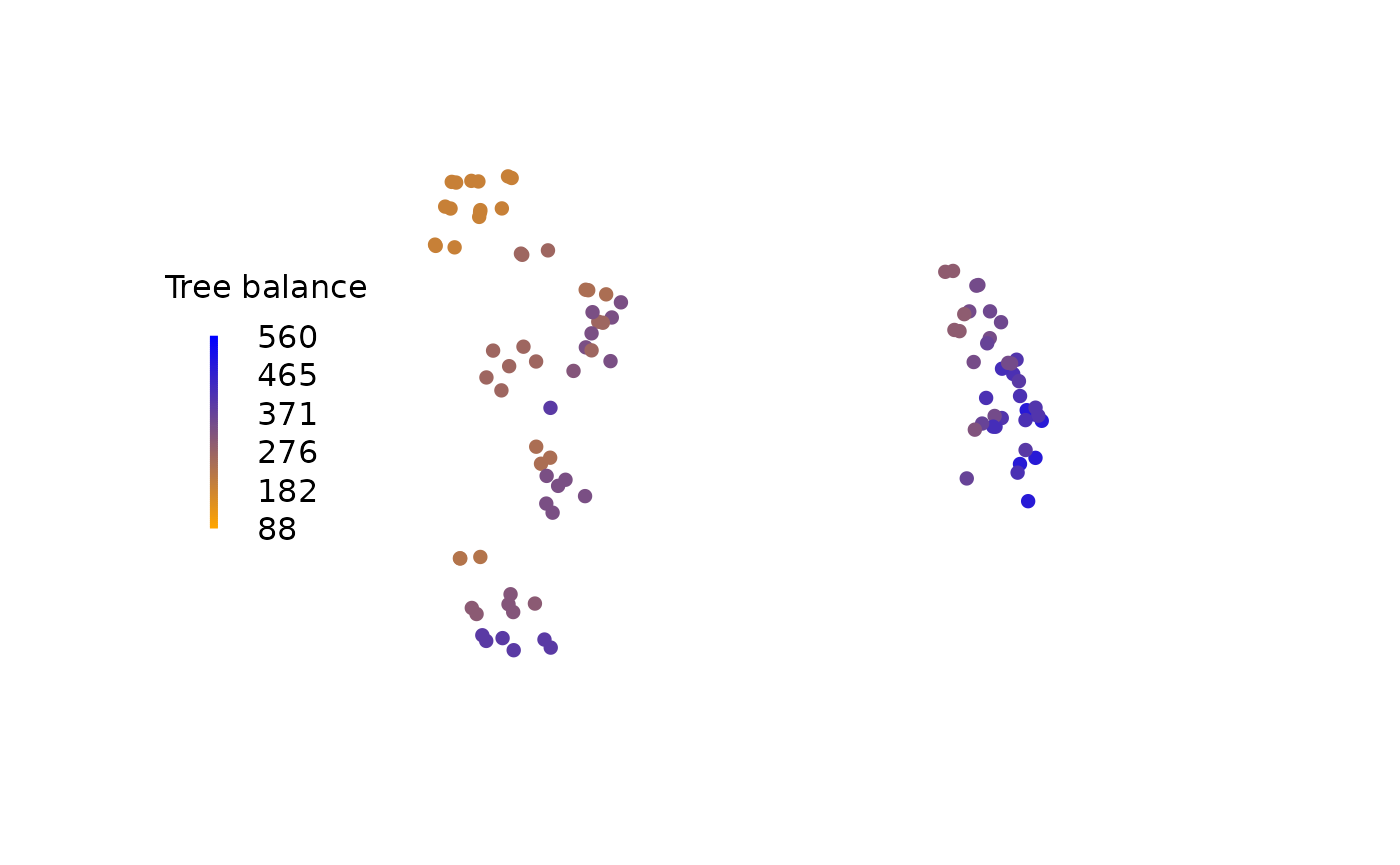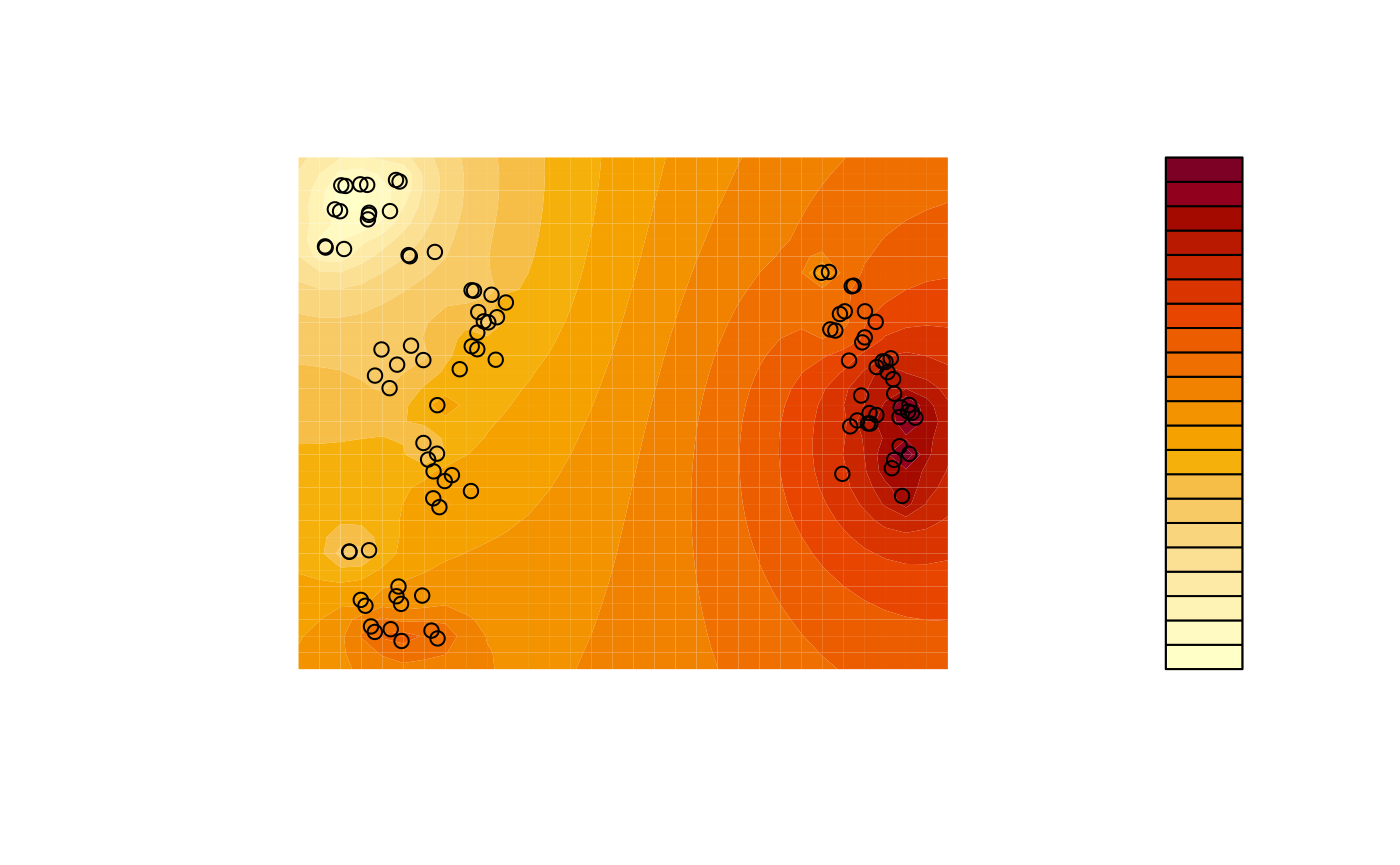Landscapes of trees are mappings of tree space that are contoured according to some optimality criterion – often, but not necessarily, a tree’s score under a phylogenetic reconstruction technique (Bastert, Rockmore, Stadler, & Tinhofer, 2002). Detecting “islands” or “terraces” of trees can illuminate the nature of the space of optimal trees and thus inform tree search strategy (Maddison, 1991; Sanderson, McMahon, & Steel, 2011).

For simplicity (and to avoid scoring trees against a dataset), this example uses a tree’s balance (measured using the total cophenetic index) as its score (Mir, Rosselló, & Rotger, 2013). We assume that mappings have already been shown to be adequate (Smith, 2022).

A landscape is most simply visualized by colouring each tree according to its score:

# Load required libraries
library("TreeTools", quietly = TRUE)
library("TreeDist")

# Generate a set of trees
trees <- as.phylo(as.TreeNumber(BalancedTree(16)) + 0:100 - 15, 16)

# Create a 2D mapping
distances <- ClusteringInfoDist(trees)
mapping <- cmdscale(distances, 2)

# Score trees according to their balance
scores <- TotalCopheneticIndex(trees)

# Normalize scores
scoreMax <- TCIContext(trees[])[["maximum"]]
scoreMin <- TCIContext(trees[])[["minimum"]]
scores <- scores - scoreMin
scores <- scores / (scoreMax - scoreMin)

# Generate colour palette
col <- colorRamp(c("orange", "blue"))(scores)
rgbCol <- rgb(col, maxColorValue = 255)

# Plot trees, coloured by their score
plot(
mapping,
asp = 1, # Preserve aspect ratio - do not distort distances
ann = FALSE, axes = FALSE, # Don't label axes: dimensions are meaningless
col = rgbCol, # Colour trees by score
pch = 16 # Plotting character: Filled circle
)

PlotTools::SpectrumLegend(
"left",
title = "Tree balance",
palette = rgb(colorRamp(c("orange", "blue"))(0:100 / 100) / 255),
legend = floor(seq(scoreMax, scoreMin, length.out = 6)),
bty = "n"
)A more sophisticated output can be produced using contours, interpolating between adjacent trees. This example uses a simple inverse distance weighting function for interpolation; more sophisticated techniques such as kriging or (in continuous tree spaces) the use of geodesics (Khodaei, Owen, & Beerli, 2022) can produce even better results.

# Use an inverse distance weighting to interpolate between measured points
Predict <- function (x, y) {
Distance <- function (a, b) {
apply(a, 2, function (pt) sqrt(colSums((pt - b) ^ 2)))
}
predXY <- rbind(x, y)
dists <- Distance(t(mapping), predXY)
invDist <- 1 / dists
weightings <- invDist / rowSums(invDist)

# Return:
colSums(scores * t(weightings))
}

# Generate grid for contour plot
resolution <- 32
xLim <- range(mapping[, 1]) * 1.1
yLim <- range(mapping[, 2]) * 1.11
x <- seq(xLim, xLim, length.out = resolution)
y <- seq(yLim, yLim, length.out = resolution)
z <- outer(x, y, Predict) # Predicted values for each grid square

# Plot
filled.contour(
x, y, z,
asp = 1, # Preserve aspect ratio - do not distort distances
ann = FALSE, axes = FALSE, # Don't label axes: dimensions are meaningless
plot.axes = {points(mapping, xpd = NA)} # Use filled.contour coordinates
)A variety of R add-on packages facilitate three-dimensional plots.

if (requireNamespace("plotly", quietly = TRUE)) {
library("plotly", quietly = TRUE)
fig <- plot_ly(x = x, y = y, z = z)
print("Run install.packages('plotly') to view this output")
}
Khodaei, M., Owen, M., & Beerli, P. (2022). Geodesics to characterize the phylogenetic landscape. bioR$$\chi$$iv. doi: 10.1101/2022.05.11.491507